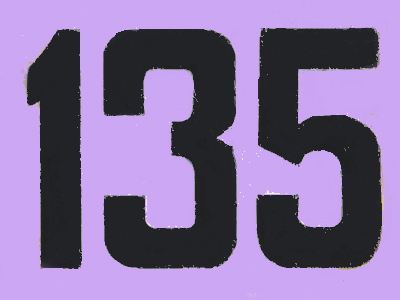The next odd number after 133 is 135.

# Number Sequences (Year 4)

As children progress through KS2 they will learn more about number sequences in Maths. As well recognising simple number sequences of increasing value they will also be exposed to decreasing sequences in more complicated steps, such as patterns decreasing by 25 each time. They will also learn more about odd and even numbers and the patterns made when adding or subtracting them from one another.

Recognising patterns in number sequences is all about finding out what comes next in a row of numbers. For instance, do you know which number should follow this sequence 20, 15, 10, 5? Well, the numbers are decreasing. or getting less, each time. Each number is 5 less than the one before it. To find the next number in the sequence we need to take 5 away from 5 which will give us the answer 0.

See how much you have learned about recognising patterns in number sequences by playing this quiz for 8-9 year olds.

1.
What is the next number of the sequence? 136, 140, 144, 148
150
152
154
156
The numbers are increasing in 4s
2.
Which is the next odd number after 151?
152
153
155
160
151 + 2 = 153
3.
Can you find the rule of this sequence? 125, 127, 129, 131, 133
The numbers increase in 2s
The numbers increase in 3s
The numbers increase in 5s
The numbers increase in 4s
The sequence would continue: 135, 137, 139, 141... etc
4.
What is the next number of the sequence? 140, 130, 120, 110
90
95
100
105
The numbers are decreasing by 10
5.
Which of these statements is true?
An even number ends in 0, 2, 4, 6 or 8
An odd number ends in 0 or 5
An even number ends in 1, 3, 5, 7 or 9
An odd number ends in 0, 2, 4, 6 or 8
Even numbers end in 0, 2, 4, 6 or 8.
Odd numbers end in 1, 3, 5, 7 or 9
6.
Can you find the rule of this sequence? 50, 75, 100, 125, 150
The numbers increase in 20s
The numbers increase in 25s
The numbers increase in 5s
The numbers increase in 10s
Each number (except the first) is 25 more than the one before it
7.
Which of these statements is true?
After 1, every third number is even
After 1, every second number is odd
Every second number starting at 1 is odd.
Every second number starting at 2 is even
8.
Can you find the rule of this sequence? 5, 8, 11, 14, 17
The numbers increase in 2s
The numbers increase in 3s
The numbers increase in 5s
The numbers increase in 10s
Each number (except the first) is 3 more than the one before it
9.
What are the next two numbers of the sequence? 4, 8, 12, 16
18, 20
20, 22
20, 24
20, 26
The numbers are increasing in 4s
10.
Can you find the rule of this sequence? 45, 35, 25, 15, 5
The numbers decrease in 2s
The numbers decrease in 3s
The numbers decrease in 5s
The numbers decrease in 10s
Each number (except the first) is 10 less than the one before it
You can find more about this topic by visiting BBC Bitesize - What is a number sequence?

Author:  Amanda Swift